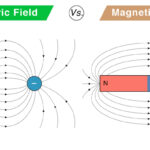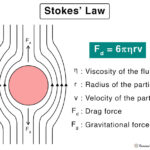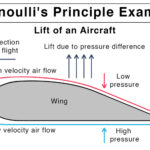Home / Physics / Normal Force

# Normal Force

## What is the Normal Force

Suppose an object is lying on a surface. It applies a force due to its weight. According to Newton’s third law of motion, the surface responds by applying an equal and opposite force on the object. The component of this force that is perpendicular to the surface is called the normal force. Since the object remains in contact with the surface, the normal force is a contact force.

The normal force balances the weight of the object. However, if other forces act on the body to displace it, friction comes into play. According to the laws of friction, the frictional force is proportional to the normal force.

## Examples of Normal Force

There are many examples of normal force, most of which take place in daily life. These include the force applied by:

• A table on a book lying on top of it
• A wedge on a block lying on its inclined surface
• Earth upon us
• Human hand while holding an object
• A nail on a hammer during hammering

## Characteristics of Normal Force

Here are some facts and properties of the normal force.

• Contact force
• Reaction force
• Perpendicular to the surface
• Opposite to the component of the object’s weight that is perpendicular to the surface

## How to Calculate Normal Force

The normal force can be calculated using physics principles and balancing the forces using Newton’s laws of motion.

### Normal Force Equations

#### 1. On a Horizontal Surface

Suppose a block of mass m is lying on a horizontal surface. Its weight W is mg, and no other forces are applied to it. The table will apply a force FN on the object. According to Newton’s third law of motion, the normal force is equal to the object’s weight. Therefore, the magnitude of FN is given by,

FN = mg

Symbol of normal force: F

Unit of normal force: Newton or N

Dimensions of normal force: MLT-2

The work done by a force is defined as the force times the displacement.

W = F x d

Since the normal force does not displace the object, so the work done is zero.

W = FN x 0 = 0

#### 2. On an Incline or Ramp

Suppose a block lies on an inclined plane that makes an angle θ with the horizontal.  Its weight mg can be resolved into two components – one parallel to the surface and the other perpendicular to the surface.

The perpendicular component is given by mg cos θ. According to Newton’s third law, this force is equal to the normal force.

FN = mg cos θ

#### 3. In an Elevator

Suppose a person of mass m is inside an elevator which is moving upward with an acceleration a. As the person is in contact with the elevator, he experiences an applied force given by,

FA = ma

Aside, the elevator also exerts a force equal to the person’s weight mg. Therefore, the total force exerted by the elevator on the person is the normal force, which is given by

FN = mg + FA

Or, FN = m(g + a)

In this situation, the normal force is greater than the weight of the person.

Suppose the elevator is moving downwards with the same acceleration, then the force experienced by the person is,

FN = m(g – a)

In this situation, the normal force is less than the weight of the person.

Now, suppose the elevator cable snaps. Then, the elevator moves downwards with an acceleration g, and the force experienced by the person is,

FN = m(g – g) = 0

Therefore, the normal force is zero, meaning that the person is not in contact with the elevator floor. This phenomenon is known as a free fall, and the person feels weightless.Electric Field vs. Magnetic FieldHooke’s LawStokes’ LawBernoulli’s Principle and Equation